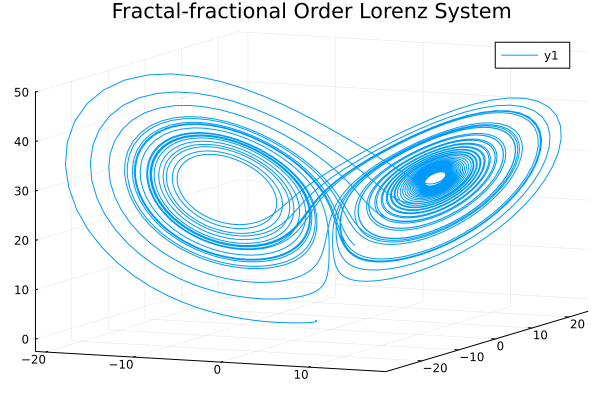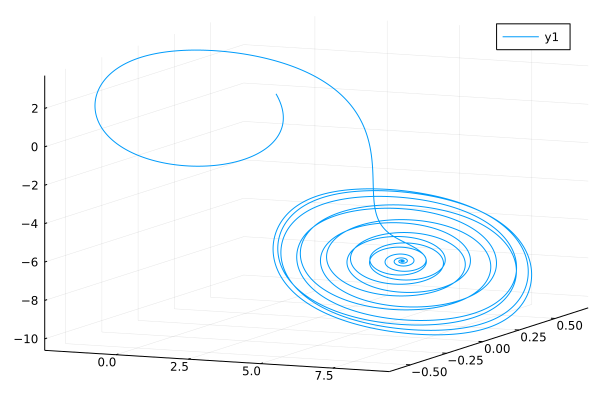# Fractal-Fractional Order Ordinary Differential Equations

The concept of fractal–fractional differentiation has appeared as a combination of two mathematical concepts: fractal differentiation and fractional differentiation. These operators are helpful for modeling more complex problems, also they allow us to understand many physical problems which have fractal properties.

Fractal-fractional derivative with power law:

$$${^{FFP}_aD^{\gamma,\theta}_t}u(t)=\frac{1}{\Gamma(1-\gamma)}\frac{d}{dt^\theta}\int_a^tu(y)(t-y)^{-\gamma}dy,\ 0<\gamma, \theta\leq1$$$

Fractal-fractional derivative with exponential decay kernel:

$$${^{FFE}_aD^{\gamma,\theta}_t}u(t)=\frac{M(\gamma)}{\Gamma(1-\gamma)}\frac{d}{dt^\theta}\int_a^tu(y)\exp(\frac{-\gamma}{1-\gamma}(t-y))dy,\ 0<\gamma, \theta\leq1$$$

Fractal-fractional derivative with Mittag Leffler kernel:

$$${^{FFM}_aD^{\gamma,\theta}_t}u(t)=\frac{AB(\gamma)}{\Gamma(1-\gamma)}\frac{d}{dt^\theta}\int_a^tu(y)E_\gamma(\frac{-\gamma}{1-\gamma}(t-y))dy,\ 0<\gamma, \theta\leq1$$$

## Fractal-fractional ODE system

To construct fractal-fractional differential problems, we need to use FFPODEProblem, FFEODEProblem and FFMODEProblem to define our problem:

julia> FFPODEProblem(f, [α, β], u0, tspan)
julia> FFEODEProblem(f, [α, β], u0, tspan)
julia> FFMODEProblem(f, [α, β], u0, tspan)

Let's see the Lorenz system in Atangana-Baleanu-Caputo sense with Mittag Leffler kernel:

$$$\begin{cases} {^{FFM}D^{\alpha,\beta}}x=a(y-x)\\ {^{FFM}D^{\alpha,\beta}}y=(b-z)x-y\\ {^{FFM}D^{\alpha,\beta}}z=xy-cz \end{cases}$$$
using FractionalDiffEq, Plots
α=1;β=1;h=0.01;tspan=(0, 50)
u0 = [-2, 1, -1]
function fun(du, u, p, t)
a=10;b=28;c=8/3
du = a*(u-u)
du = (b-u)*u-u
du = u*u-c*u
end
prob = FFODEProblem(fun, [α, β], u0, tspan)
sol = solve(prob, h, AtanganaSeda())
plot3d(sol, vars=(1,2,3) title="Fractal-fractional Order Lorenz System")## Variable order fractal-fractional ODE problem

using FractionalDiffEq, Plots

alpha=0.96;h=0.01;tfinal=100;
β(t) = 0.01+0.01*t
u0=[-0.2; 0.5; 0.2]
function fun(du, u, p, t)
gama=10.814;lambda=14;a=0.2;b=0.15;
du = gama*(u-a*sin(2*pi*b*u))
du = u-u+u
du = -lambda*u
end

prob = FFODEProblem(fun, [alpha, β], u0, (1, tfinal))
sol = solve(prob, h, AtanganaSeda())
plot3d(sol, vars=(1,2,3))